# 光谱相机探测水体污染物可行性研究Feasibility Study of Spectral Camera for Detecting Water Pollutants

DOI: 10.12677/APP.2020.1012069, PDF, HTML, XML, 下载: 87  浏览: 158

Abstract: Spectral imaging technology plays an irreplaceable role in agriculture, mineral identification, plant ecology, food safety, medical diagnosis, aerospace and other fields due to its high accuracy, high resolution, continuity, remote sensing and other advantages, and has aroused the interest of many researchers due to its wide application prospect. In this paper, the principle of spectral camera and its application in water quality detection by remote sensing technology are described, in order to study the feasibility of spectral camera in detecting water pollutants and the quality of detection results, simulation experiments are designed and carried out. After image analysis, it is found that the simulated sewage has the highest reflectance in the green light band, but not particularly high in the red and blue light bands. In contrast, the reflection in the red band is better than that in the blue band. At the same time, the best observation point is found through the calculation results of gray mean value. It can be used as a reference for monitoring water pollution by spectral camera in practice.

1. 引言

2. 光谱相机及光谱分析的技术原理

2.1. 光谱相机

20世纪80年代，第一台成像光谱仪在美国被研制出来。光谱相机拥有多光谱相机、高光谱相机、超光谱相机等多种类型，其中多光谱相机可以在红外、近红外、紫外光等范围进行拍摄，利用滤光片、分光镜等设备对光线进行过滤、分解，可以在感光胶片上形成目标在不同波长的光波下的光谱图片，而且图片分辨率较高。光谱相机的基本结构由图像采集系统、光学系统、控制与信息处理器、探测器等装置组成。

2.2. 双向反射分布函数

BRDF最早是在光辐射角度进行定义，是用来描述光线如何在物体表面进行反射，表现其空间反射分布特性和光谱特性，其理论认为一个表面被一个方向光照射后会发生反射，但是在表面上方整个半球的每个方向都会有反射光出射，各个方向上反射的能量是不同的，BRDF表示的某个确定反射方向的光与指定方向入射光的关系：

$f\left(l,v\right)=\frac{d{L}_{0}\left(v\right)}{d{E}_{l}}$ (2-1)

$\text{Φ}=\frac{dQ}{dt}$ (2-2)

E表示的是辐射照度是指受照面单位面积上的辐射通量，定义如下：

$E=\frac{d\text{Φ}}{dA}$ (2-3)

L为辐射率，指的是某单位面积内的单位立体角的辐射通量密度，其中 $d{A}^{\perp }$ 是面积微分dA在与光线垂直方向上的投影，如下图1

$L=\frac{d\text{Φ}}{d\omega d{A}^{\perp }}$ (2-4)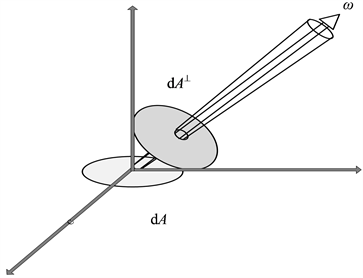Figure 1. Differential area projection

BRDF也可以用表面上半球建立球坐标系来表示，如下图2θ为极角是指入射或反射光线与法线的夹角，同时在平面上建立一个坐标轴，入射反射光线在平面上投影与该坐标轴的夹角为ϕ，称为方位角。由此BRDF也可以用极角和方位角表示： $f\left({\theta }_{i},{\varphi }_{i},{\theta }_{o},{\varphi }_{o}\right)$，对于各向同性的物质，可以用两光线投影的夹角ϕ来代替 ${\varphi }_{i}$${\varphi }_{o}$，即 $f\left({\theta }_{i},{\theta }_{o},\varphi \right)$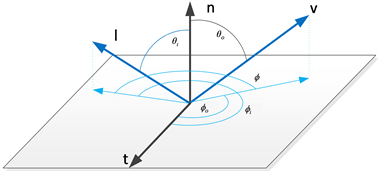Figure 2. Spherical coordinate system

3. 污水检测实验

3.1. 实验准备

3.2. 实验内容、方法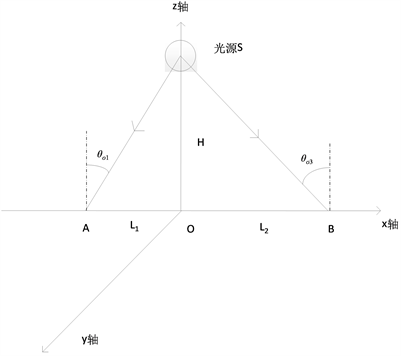Figure 3. Schematic diagram of simulation experiment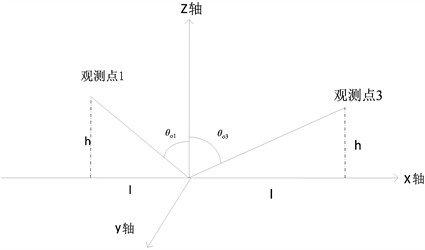Figure 4. Two observations in the XOZ plane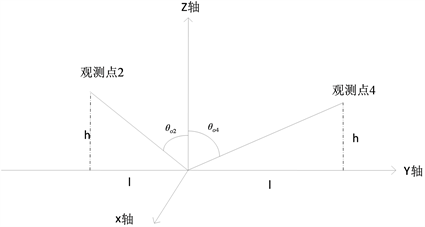Figure 5. Two observations in the YOZ plane

3.3. 图像采集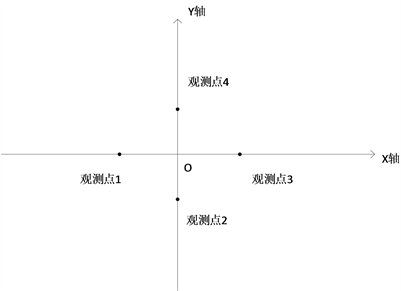Figure 6. The projection of four observation points at O onto the XOY planeTable 1. Fature picture taken at observation point 1 at point ATable 2. Fature picture taken at observation point 2 at point ATable 3. Fature picture taken at observation point 3 at point ATable 4. Fature picture taken at observation point 4 at point ATable 5. Fature picture taken at observation point 5 at point OTable 6. Fature picture taken at observation point 2 at point OTable 7. Fature picture taken at observation point 3 at point OTable 8. Fature picture taken at observation point 4 at point OTable 9. Fature picture taken at observation point 1 at point BTable 10. Fature picture taken at observation point 2 at point BTable 11. Fature picture taken at observation point 3 at point BTable 12. Fature picture taken at observation point 4 at point B

4. 数据分析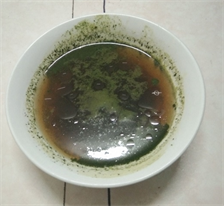Figure 7. An image taken without a filter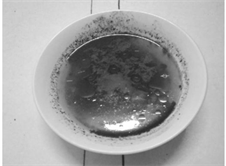Figure 8. Using MATLAB to extract the red image, through the red filter image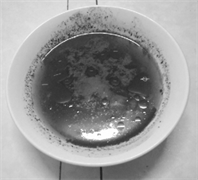Figure 9. Using MATLAB to extract the green image, through the green filter image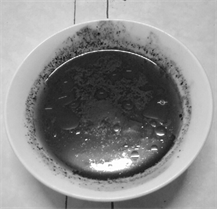Figure 10. Using MATLAB to extract the blue image, through the blue filter imageTable 13. Gray level and BRDF representation of A, O and B points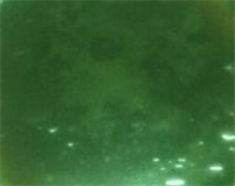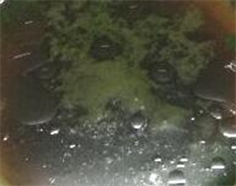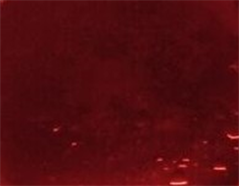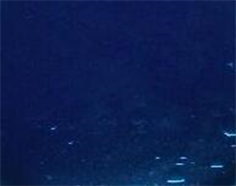Figure 11. Four captured sewage images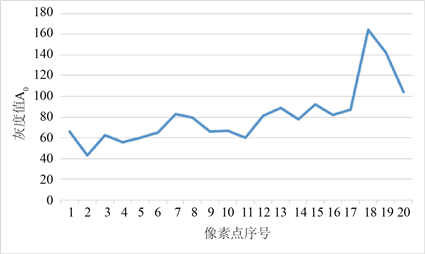Figure 12. The gray value of a 20-pixel image without filter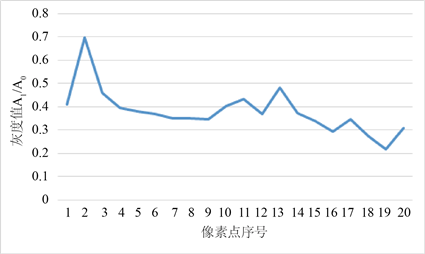Figure 13. Gray value of normalized blue filter image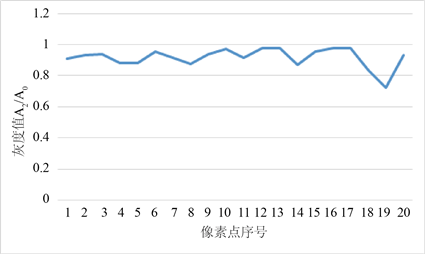Figure 14. Gray value of normalized green filter image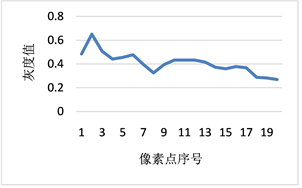Figure 15. Gray value of normalized red filter image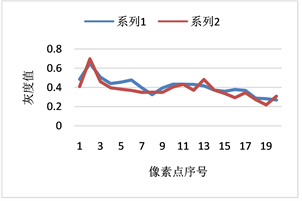Figure 16. Comparison of gray values of red and blue filter images

5. 结论

NOTES

*通讯作者。

  许洪, 王向军. 多光谱，超光谱成像技术在军事上的应用[J]. 红外与激光工程, 2007, 36(1): 13-17.  中华人民共和国生态环境部. 2018年中国海洋生态环境状况公报[R]. 2019.  Gonzalez, C., Quevaviller, P. and Green-wood, R. (2009) Rapid Chemical and Biological Techniques for Water Monitoring. John Wiley & Sons Ltd., Chichester. https://doi.org/10.1002/9780470745427  Manobianco, J. ( 2004) NSTI Nanotech. 1, 422.  Thomas, O. (2007) UV Visible Spectrophotometry of Water and Wastewater. Elsevier, Oxford.  董平敏. 遥感技术在水文水资源领域中的应用分析[J]. 中国地名, 2019(5): 37.  中国科学院环境科学委员会, 天津市环境保护局. 天津-渤海湾地区环境遥感论文集[C]. 北京: 科学出版社, 1985.  疏小舟, 汪骏发. 航空成像光谱水质遥感研究[J]. 红外与毫米波学报, 2000, 19 (4): 273-276.  马荣华, 戴锦芳. 应用实测光谱估测太湖梅梁湾附近水体叶绿素浓度[J]. 遥感学报, 2005(1): 78-86.  段洪涛, 张寿选, 张渊智. 太湖蓝藻水华遥感监测方法[J]. 湖泊科学, 2008(2): 145-152.  孙鑫, 白加光, 王忠厚, 白清兰, 黄旻. 一种机载多光谱相机的光学系统设计[J]. 光子学报, 2009, 38(12): 3160-3164.  赵峰. 一种用于海洋赤潮监测的多光谱高分辨相机设计[D]: [硕士学位论文]. 哈尔滨: 哈尔滨工业大学, 2019.